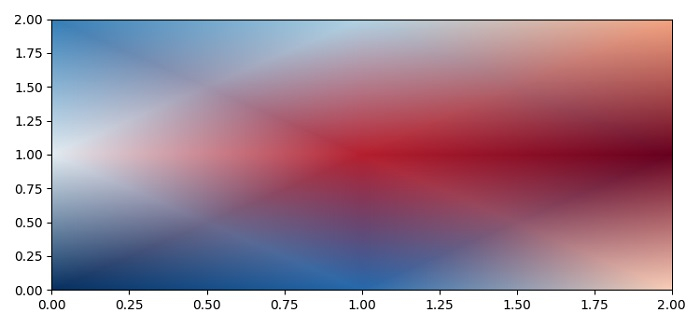# How to get smooth interpolation when using pcolormesh (Matplotlib)?

To get smooth interpolation when using pcolormesh, we can use shading="gouraud" class by name.

## Steps

• Set the figure size and adjust the padding between and around the subplots.

• Create data, x and y using numpy meshgrid.

• Create a pseudocolor plot with a non-regular rectangular grid using pcolormesh() method.

• To display the figure, use show() method.

## Example

import matplotlib.pylab as plt
import numpy as np

plt.rcParams["figure.figsize"] = [7.50, 3.50]
plt.rcParams["figure.autolayout"] = True

data = np.random.random((3, 3))
x = np.arange(0, 3, 1)
y = np.arange(0, 3, 1)
x, y = np.meshgrid(x, y)

plt.show()